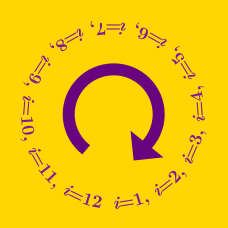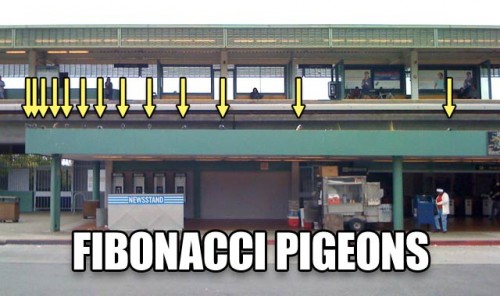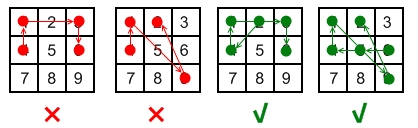Computer Science

# Loops: Level 4 Challenges

There are p knights sitting at positions $[1, 2, ... p]$ at King Arthur's round table. In order to choose the leader, Arthur starts counting at position 1 and dismisses every rth seated knight until only 1 knight remains. The remaining seated knight becomes the leader.

Let $C(p, r)$ be the integer position of the eventual leader when the table starts out with p knights and every rth seated one is dismissed.

Let T = $C(999,11) + C(121, 12) + C(2, 1) + C(15, 16) + C(99, 3)$. What is T?

Details and assumptions

$C(4, 2) = 1$. The knights start seated at positions 1, 2, 3, 4. Arthur dismisses them in this order: 2, 4, 3. The knight seated at position 1 becomes the leader.I guess you all familiar with the Fibonacci sequence.

For those who haven't known this term yet, the Fibonacci sequence is a sequence of numbers which $F(0)=0$, $F(1)=1$ and for $n \ge 2$, $F(n)=F(n-1)+F(n-2)$.

So, here's my question:

If $F(x)$ is the largest prime Fibonacci number where $x \le 200$, find $\left\lfloor \log _{ x }{ F(x) } \right\rfloor$.

###### This problem belongs to this set

Android's lock screen feature allows people to protect their phones by joining points on a grid to make a pattern. Let $L$ be the size of the longest possible length of the pattern that can be drawn on an android phone with a $3 \times 3$ pattern lock. If the grid is spaced equally by one unit what is $L \sqrt{31}$ rounded to the nearest integer?

The rules for drawing a pattern are:

• You can only use a point once

• You cannot "jump" a point if it is in between the two points and is part of the line that satisfies the first two points. Ie You cannot go from 1 to 9 without also hitting 5. But you can go directly from 2 to 9 without including any other point.

• ONCE a point is "taken", it may THEN be skipped. A point cannot be skipped "in transit" only if it hasn't already been taken.

To fully understand the rules, it is best to try them out on a real phone.Details and Assumptions

• The points are equally spaced vertically and horizontally by one unit.

• If it had been a $2 \times 2$ grid, $L$ would be $1 + 2 \sqrt{2}$.

×